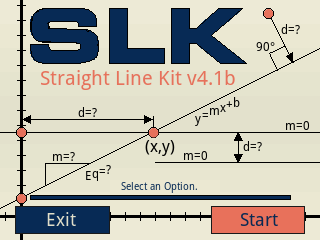## SLK (Straight Line Kit)

Description:Helps you to find the distance, the slope and the equation between two points, the perpendicular distance between a point and a straight line, collinearity of three points (the middle-point and the end-point coordinates), the equation of a straight line that goes through a point with a slope, transforms linear equations back and forth between standard and slope-intercept forms, generates the coordinate representation of a straight line, determines whether two straight lines are parallel or perpendicular, finds the (x) & (y) intercepts of a straight line, finds the intersection point of 2 straight lines, transforms a non-standard form equation into a standardized form of it, divide a segment into points or sub-segments, performs linear interpolation/extrapolation. Requires firmware 10077 or higher.
Filename:slk41b.zip
ID:7575
Current version:4.1b
Author:Dante Camargo
Size on calculator:280 KB
Platforms:Prime
User rating:10/10 with 1 vote (you must be logged in to vote)
Primary category:Math
Languages:ENG ESP
File date:2018/09/07 23:58:31
Creation date:2018/09/07
Source code:Not included
Version history:2018/09/09: Updated to version 4.1b
2017/02/10: Updated to version 4.1
2016/08/02: Updated to version 3.3
Screenshot: